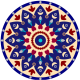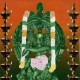# Modulo rugs of 3D functionsmAnasa-taraMgiNI

Consider a 3D function \$latex z=f(x,y)\$. Now evaluate it at each point of a \$latex n times n\$ integer lattice grid. Compute \$latex z mod n\$ corresponding to each point and plot it as a color defined by some palette that suits your aesthetic. The consequence is a what we term the “modulo rug”.
For example, below is a plot of \$latex z=x^2+y^2\$.Figure 1: \$latex z=x^2+y^2, n=318\$

We get a pattern of circles around a central circular system reminiscent of ogdoadic arrangements in various Hindu maṇḍala-s. From the aesthetic viewpoint, the best modulo rugs are obtained with symmetric functions higher even powers — this translates into some pleasing symmetry in the ru. Several examples of such are shown below.Figure 2: \$latex z=x^4-x^2-y^2+y^4, n=318\$Figure 3: \$latex z=x^4-x^2-y^2+y^4, n=315\$Figure 4: \$latex z= x^6-x^4-y^4+y^6, n=309\$Figure 5: \$latex z=x^6-x^2-y^2+y^6, n=318\$Figure 6: \$latex z=x^4-x^2+y^2-y^4, n=310\$

All the above \$latex…

View original post 51 more words